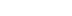/
article

# Lone Game Developer Battles Physics Simulator

A real-time 3D graphics developer needs to wage many battles. In this article he documents one of the less glamorous battles — the battle of the physics simulator. It’s not going to be fun. It’s going to be a bit bloody. However, if a developer ever hopes to achieve a realistic and interesting physics simulation, it’s a battle that must be fought.

As a real-time 3D graphics developer, I need to wage many battles. I fight with artists over polygon counts, with graphics card manufacturers over incomplete or incorrect drivers, and with some producers’ tendencies to continuously expand feature lists. However, some of the greatest battles I have fought have been with myself. I fight to bring back the knowledge I have long since forgotten. I fight my desire to play the latest action game when more pressing needs are at hand (deadlines, the semblance of a social life).

In this I document one of the less glamorous battles — the battle of the physics simulator. It’s not going to be fun. It’s going to be a bit bloody. However, if I ever hope to achieve a realistic and interesting physics simulation, it’s a battle that must be fought. So, my brave warriors, join me. Sharpen your pencils, stock your first-aid kit with plenty of aspirin, drag out the calculus book, and fire up the coffeepot. Let’s get started.

I hope you all had a chance to play around with the soft body dynamics simulator from my article titled Collision Response: Bouncy, Trouncy Fun. The demo highlighted an interesting problem — the need for stability. While creating my dynamics simulation, I waged a constant battle for stability. However, in order to wage the war effectively, I need to understand the roots of the instability in the system. Last month, I implied that the problem resulted from my use of a simple Euler integrator. But I didn’t really explain why that caused the problem. Let me fix that right now.

Integrators and You

Many game programmers never realize that when they create the physics model for their game, they are using differential equations. One of my first programs on the Apple II was a spaceship flying around the screen. My "physics" loop looked like this:

ShipPosition = ShipPosition + ShipVelocity;

ShipVelocity = ShipVelocity + ShipAcceleration;

Look familiar to anyone? It’s a pretty simple physics model, but it turns out that even here I was integrating. If you look at the Euler integrator from last month, I had

Position = Position + (DeltaTime * Velocity);

Velocity = Velocity + (DeltaTime * Force * OneOverMass);

Now for my simple physics model, DeltaTime = 1 and Mass = 1. Guess what? I was integrating with Euler’s method and didn’t even know it. If I had made this Apple II physics model any more complex, this integrator could have blown up on me. These sorts of problems can be difficult to track down, so it’s important to understand the causes.

When Things Go Wrong

The reason that the Euler integrator can blow up is that it’s an approximation. I’m trying to solve a differential equation by using an iterative numerical method. The approximation can differ from the true value and cause error. When this error gets too large, the simulation can fail. A concrete example may help to explain. Last month, I added a viscous drag force to the simulation to add stability. The formula for this force was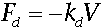(Eq. 1)

In this formula, kd represents the coefficient of drag that is multiplied by the velocity of the particle. This coefficient determines how fast the velocity of the object is dragged down to zero. This is a very simple differential equation. In fact, it’s simple enough to be satisfied for v directly by the formula. I can use this exact solution to check the accuracy of my numerical integrator: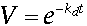(Eq. 2)

Euler’s method is used to approximate the integral curve of Equation 2 with a series of line segments along this path. Each step along this path is taken every time, interval h, via the formula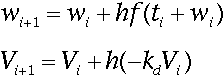(Eq. 3)

In all cases, the viscous drag force should approach zero. However, the size of the step h and coefficient of drag kd determine how well the approximation performs. Take a look at Figure 1.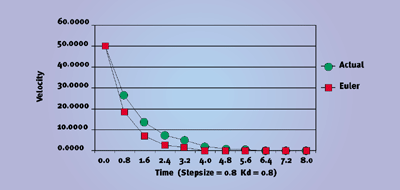Figure 1. A decent approximation.

With the given step size and drag coefficient, Euler’s method may not be a great approximation, but it gives the desired result. The velocity converges on zero. But take a look at the relationship between the step size and drag coefficient in Equation 3.

If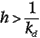then the approximation step will overshoot zero, as you can see in Figure 2.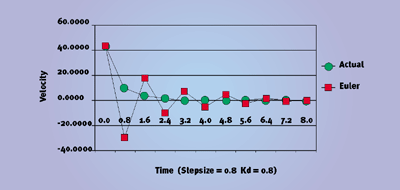Figure 2. This looks a lot worse.

By increasing the step size, I was trying to get a system that converged to zero more quickly — but I got something entirely different. Things really start to get bad when the drag coefficient increases more, as in Figure 3. As each step is taken, not only does the approximation oscillate across zero, but it also actually diverges from zero, and eventually explodes the system. This is exactly what was happening in the spring demonstration from last month, when the box blew up.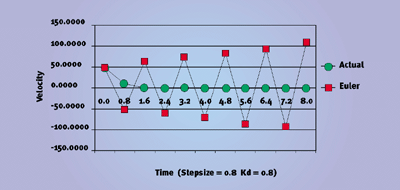Figure 03. Kaboom!

How Can I Prevent Explosions?

If you find a situation where your simulator blows up, there’s an easy way to see if this kind of numerical instability is the cause. Reduce the step size. If you reduce the size of the step and the simulation works, then this numerical instability is the problem.

The easy solution is always to take small steps. However, realize that each step requires quite a few calculations. The simulation will run faster if it can take fairly large step sizes. Unfortunately, when you get lots of objects interacting, these instability problems appear even more. So, just when things start to get interesting, you need to reduce the step size and slow things down.

I’d rather create an integrator that would allow me to take large step sizes without sacrificing stability. To do this, I need to look at the origins of Euler’s method.

Taylor’s Theorem

You may remember Taylor’s Theorem from calculus. It’s named after mathematician Brook Taylor’s work in the eighteenth century. This theorem describes a method for converging on the solution to a differential equation.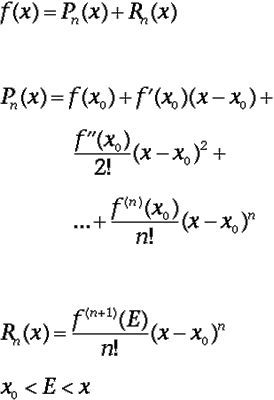(Eq. 4)

In Equation 4, Pn(x) represents the nth Taylor polynomial. If you take the limit of Pn(x) as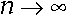you get the Taylor series for the function. If, however, the infinite series is not calculated and the series is actually truncated, Rn(x) represents the error in the system. This error is called the truncation error of approximation.

How does this apply to the problem with which we are working? If I only look at the first Taylor polynomial and do some substitution, I get Equation 5.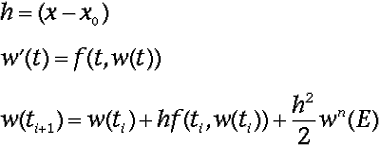(Eq. 5)

Notice how similar this equation is to Equation 3. In fact, Euler’s method is based on this equation. The only difference is that the last error term is dropped in Equation 5. By stopping the series at the second term, I get a truncation error of 2. This gives Euler’s method an error of order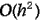.

If I added another term of the Taylor series to the equation, I could reduce the error to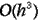. However, to compute this exactly, I would need to evaluate the next derivative of f(x). To avoid this calculation, I can do another Taylor expansion and approximate this derivative as well. While this approximation increases the error slightly, it preserves the error bounds of the Taylor method. This method of expansion and substitution is known as the Runge-Kutta techniques for solving differential equations. This first expansion beyond Euler’s method is known as the Midpoint method or RK2 (Runge-Kutta order 2), and is given in Equation 6. It’s called the Midpoint method because it uses the Euler approximation to move to the midpoint of the step, and evaluates the function at that new point. It then steps back and takes the full time step with this midpoint approximation.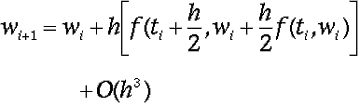(Eq. 6)

In fact, I can continue to add Taylor terms to the equation using the Runge-Kutta technique to reduce the error further. Each expansion requires more evaluations per step, so there is a point at which the calculations outweigh the benefit. I don’t have the space to get into it here, however, I understand that smaller step sizes are preferred over methods above RK4 with an error of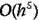(Faires & Burden, p. 195). Runge-Kutta order 4 is outlined in Equation 7.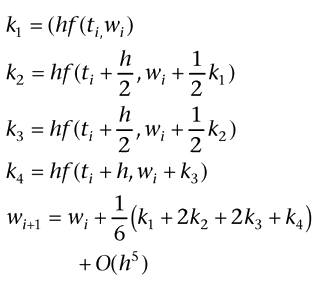(Eq. 7)

RK4 gives the simulation a very robust integrator. It should be able to handle most situations without blowing up. The only issue now is what the step size should be.

Even with a robust integrator such as RK4, there will be times when the simulation will be in danger of blowing up. To keep this from happening, you may have to reduce the step size at times. At other times, however, a large step size works fine. If my simulator only has a single fixed step size, I cannot take advantage of these facts. If I vary the size of the steps according to need, I could use large steps when possible without sacrificing stability.

This is how it works. I take full step using my current integrator, then take two steps half the current step size, and compare the results. If the error between the two results is greater than a threshold, then the step size should be reduced. Conversely, if the error is less than the threshold, the step size could actually be increased. This form of control is known as an adaptive step size method. Adaptive methods are a major area of research in numerical analysis, and can definitely improve simulation performance. I chose not to implement adaptive step size controls in my simulation. However, this is an area where you could improve the simulation.

Other Techniques

Differential equations are not easy to learn and understand. However, the programmer who pursues this knowledge has many weapons in his arsenal. As witnessed by the birthdates of Euler and Taylor, this research has been going on for centuries. If you ignore this work and strike out on your own, you’re doing yourself a great disservice. Knowledge is available to the developer as never before. While working on these algorithms, I was able to cross-check formulas and techniques in many different sources.

In fact, I’ve barely scratched the surface of the field. The integrators I’ve described (all explicit one-step methods) represent only a subset of the methods available to the programmer. Implicit integrators will also work. For example, an implicit Runge-Kutta integrator trades greater computations per step for greater stability in particularly difficult differential equations. Also, the one-step nature of these integrators reflects the fact that the method does not consider any trends in the past when calculating a new value.

In addition to these one-step methods, there are also multistep methods, extrapolation algorithms, predictor-corrector methods, and certainly many others. Clearly, there is plenty of ground for the adventurous programmer to explore. The book I used, Numerical Algorithms with C, does a good job of comparing different methods during a variety of test conditions.

For this month’s sample application (available from Game Developer’s web site), I have implemented both the midpoint method and Runge-Kutta order 4 in the dynamic simulation from last month. You can switch between integrators and adjust the step size and simulation variables to get a feel for how each performs.

For Further Info

In addition to the references cited last month, a couple of other sources proved very valuable during this article.

• Faires, J. Douglas and Richard Burden. Numerical Methods. Second edition. Pacific Grove, California: Brooks/Cole, 1998. This book provided a great discussion of measuring error in numerical solutions. It also contains a great deal of source code for all the algorithms.

• Engeln-Müllges, Gisela and Frank Uhlig. Numerical Algorithms with C. , New York, New York: Springer-Verlag, 1996. In addition to the fine sections on the methods discussed in this column, this book describes and compares a great number of other numerical methods. Additionally, the book has a great number of references to articles on the topic.

• Press, William H. et al., Numerical Recipes in C. Cambridge, England: Cambridge University Press, 1998.

While not as strong a reference on these topics, this book may be interesting to many, as it is available in electronic form. See http://www.nr.com but also check out a critical discussion of it on http://math.jpl.nasa.gov/nr/nr.html.

Jeff Lander is the technical director of Darwin 3D where he spends time calculating his rate of procrastination with respect to his articles. E-mail optimization suggestions to [email protected].

### Latest Jobs

#### Treyarch

Playa Vista, California
6.20.22
Audio Engineer

#### Digital Extremes

6.20.22
Communications Director

6.20.22
Senior Producer

#### Build a Rocket Boy Games

Edinburgh, Scotland
6.20.22
More Jobs